# Sky maps (DL4)#

`gammapy.maps` contains classes for representing pixelized data structures with at least two spatial dimensions representing coordinates on a sphere (e.g. an image in celestial coordinates). These classes support an arbitrary number of non-spatial dimensions and can represent images (2D), cubes (3D), or hypercubes (4+D). Multiple pixelization schemes are supported:

• WCS : Projection onto a 2D cartesian grid following the conventions of the World Coordinate System (WCS). Pixels are square in projected coordinates and as such are not equal area in spherical coordinates.

• HEALPix : Hierarchical Equal Area Iso Latitude pixelation of the sphere. Pixels are equal area but have irregular shapes. Documentation specific to HEALPix-based maps is provided in HEALPix-based maps.

• Region : A single spatial pixel with arbitrary shape defined by a region on the sky. Documentation specific to region-based maps is provided in RegionGeom and RegionNDMap.

`gammapy.maps` is organized around two data structures: geometry classes inheriting from `Geom` and map classes inheriting from `Map`. A geometry defines the map boundaries, pixelization scheme, and provides methods for converting to/from map and pixel coordinates. A map owns a `Geom` instance as well as a data array containing map values. Where possible it is recommended to use the abstract `Map` interface for accessing or updating the contents of a map as this allows algorithms to be used interchangeably with different map representations. The following reviews methods of the abstract map interface.

## Getting started with maps#

All map objects have an abstract interface provided through the methods of the `Map`. These methods can be used for accessing and manipulating the contents of a map without reference to the underlying data representation (e.g. whether a map uses WCS or HEALPix pixelization). For applications which do depend on the specific representation one can also work directly with the classes derived from `Map`. In the following we review some of the basic methods for working with map objects, more details are given in the Maps tutorial.

## Accessor methods#

All map objects have a set of accessor methods provided through the abstract `Map` class. These methods can be used to access or update the contents of the map irrespective of its underlying representation. Four types of accessor methods are provided:

• `get` : Return the value of the map at the pixel containing the given coordinate (`get_by_idx`, `get_by_pix`, `get_by_coord`).

• `interp` : Interpolate or extrapolate the value of the map at an arbitrary coordinate.

• `set` : Set the value of the map at the pixel containing the given coordinate (`set_by_idx`, `set_by_pix`, `set_by_coord`).

• `fill` : Increment the value of the map at the pixel containing the given coordinate with a unit weight or the value in the optional `weights` argument (`fill_by_idx`, `fill_by_pix`, `fill_by_coord`).

Accessor methods accept as their first argument a coordinate tuple containing scalars, lists, or numpy arrays with one tuple element for each dimension of the map. `coord` methods optionally support a `dict` or `MapCoord` argument.

When using tuple input the first two elements in the tuple should be longitude and latitude followed by one element for each non-spatial dimension. Map coordinates can be expressed in one of three coordinate systems:

• `idx` : Pixel indices. These are explicit (integer) pixel indices into the map.

• `pix` : Coordinates in pixel space. Pixel coordinates are continuous defined on the interval [0,N-1] where N is the number of pixels along a given map dimension with pixel centers at integer values. For methods that reference a discrete pixel, pixel coordinates will be rounded to the nearest pixel index and passed to the corresponding `idx` method.

• `coord` : The true map coordinates including angles on the sky (longitude and latitude). This coordinate system supports three coordinate representations: `tuple`, `dict`, and `MapCoord`. The tuple representation should contain longitude and latitude in degrees followed by one coordinate array for each non-spatial dimension.

The coordinate system accepted by a given accessor method can be inferred from the suffix of the method name (e.g. `get_by_idx`). The following demonstrates how one can access the same pixels of a WCS map using each of the three coordinate systems:

```from gammapy.maps import Map

m = Map.create(binsz=0.1, map_type='wcs', width=10.0)

vals = m.get_by_idx( ([49,50],[49,50]) )
vals = m.get_by_pix( ([49.0,50.0],[49.0,50.0]) )
vals = m.get_by_coord( ([-0.05,-0.05],[0.05,0.05]) )
```

Coordinate arguments obey normal numpy broadcasting rules. The coordinate tuple may contain any combination of scalars, lists or numpy arrays as long as they have compatible shapes. For instance a combination of scalar and vector arguments can be used to perform an operation along a slice of the map at a fixed value along that dimension. Multi-dimensional arguments can be use to broadcast a given operation across a grid of coordinate values.

```import numpy as np
from gammapy.maps import Map

m = Map.create(binsz=0.1, map_type='wcs', width=10.0)
coords = np.linspace(-4.0, 4.0, 9)

# Equivalent calls for accessing value at pixel (49,49)
vals = m.get_by_idx( (49,49) )
vals = m.get_by_idx( (,) )
vals = m.get_by_idx( (np.array(), np.array()) )

# Retrieve map values along latitude at fixed longitude=0.0
vals = m.get_by_coord( (0.0, coords) )
# Retrieve map values on a 2D grid of latitude/longitude points
vals = m.get_by_coord( (coords[None,:], coords[:,None]) )
# Set map values along slice at longitude=0.0 to twice their existing value
m.set_by_coord((0.0, coords), 2.0*m.get_by_coord((0.0, coords)))
```

The `set` and `fill` methods can both be used to set pixel values. The following demonstrates how one can set pixel values:

```from gammapy.maps import Map
import numpy as np

m = Map.create(binsz=0.1, map_type='wcs', width=10.0)

m.set_by_coord(([-0.05, -0.05], [0.05, 0.05]), [0.5, 1.5])
m.fill_by_coord( ([-0.05, -0.05], [0.05, 0.05]), weights=np.array([0.5, 1.5]))
```

## Interface with MapCoord and SkyCoord#

The `coord` accessor methods accept `dict`, `MapCoord`, and `SkyCoord` arguments in addition to the standard `tuple` of `ndarray` argument. When using a `tuple` argument a `SkyCoord` can be used instead of longitude and latitude arrays. The coordinate frame of the `SkyCoord` will be transformed to match the coordinate system of the map.

```import numpy as np
from astropy.coordinates import SkyCoord
from gammapy.maps import Map, MapCoord, MapAxis

lon = [0, 1]
lat = [1, 2]
energy = [100, 1000]
energy_axis = MapAxis.from_bounds(100, 1E5, 12, interp='log', name='energy')

skycoord = SkyCoord(lon, lat, unit='deg', frame='galactic')
m = Map.create(binsz=0.1, map_type='wcs', width=10.0,
frame="galactic", axes=[energy_axis])

m.set_by_coord((skycoord, energy), [0.5, 1.5])
m.get_by_coord((skycoord, energy))
```

A `MapCoord` or `dict` argument can be used to interact with a map object without reference to the axis ordering of the map geometry:

```coord = MapCoord.create(dict(lon=lon, lat=lat, energy=energy))
m.set_by_coord(coord, [0.5, 1.5])
m.get_by_coord(coord)
m.set_by_coord(dict(lon=lon, lat=lat, energy=energy), [0.5, 1.5])
m.get_by_coord(dict(lon=lon, lat=lat, energy=energy))
```

However when using the named axis interface the axis name string (e.g. as given by `MapAxis.name`) must match the name given in the method argument. The two spatial axes must always be named `lon` and `lat`.

## MapCoord#

`MapCoord` is an N-dimensional coordinate object that stores both spatial and non-spatial coordinates and is accepted by all `coord` methods. A `MapCoord` can be created with or without explicitly named axes with `MapCoord.create`. Axes of a `MapCoord` can be accessed by index, name, or attribute. A `MapCoord` without explicit axis names can be created by calling `MapCoord.create` with a `tuple` argument:

```import numpy as np
from astropy.coordinates import SkyCoord
from gammapy.maps import MapCoord

lon = [0.0, 1.0]
lat = [1.0, 2.0]
energy = [100, 1000]
skycoord = SkyCoord(lon, lat, unit='deg', frame='galactic')

# Create a MapCoord from a tuple (no explicit axis names)
c = MapCoord.create((lon, lat, energy))
print(c, c['lon'], c.lon)
print(c, c['lat'], c.lat)
print(c, c['axis0'])

# Create a MapCoord from a tuple + SkyCoord (no explicit axis names)
c = MapCoord.create((skycoord, energy))
print(c, c['lon'], c.lon)
print(c, c['lat'], c.lat)
print(c, c['axis0'])
```

The first two elements of the tuple argument must contain longitude and latitude. Non-spatial axes are assigned a default name `axis{I}` where `{I}` is the index of the non-spatial dimension. `MapCoord` objects created without named axes must have the same axis ordering as the map geometry.

A `MapCoord` with named axes can be created by calling `MapCoord.create` with a `dict`:

```c = MapCoord.create(dict(lon=lon, lat=lat, energy=energy))
print(c, c['lon'], c.lon)
print(c, c['lat'], c.lat)
print(c, c['energy'])

c = MapCoord.create({'energy': energy, 'lon': lon, 'lat': lat})
print(c, c['energy'])
print(c, c['lon'], c.lon)
print(c, c['lat'], c.lat)

c = MapCoord.create(dict(skycoord=skycoord, energy=energy))
print(c, c['lon'], c.lon)
print(c, c['lat'], c.lat)
print(c, c['energy'])
```

Spatial axes must be named `lon` and `lat`. `MapCoord` objects created with named axes do not need to have the same axis ordering as the map geometry. However the name of the axis must match the name of the corresponding map geometry axis.

## Using gammapy.maps#

### Examples using `gammapy.maps.WcsNDMap`#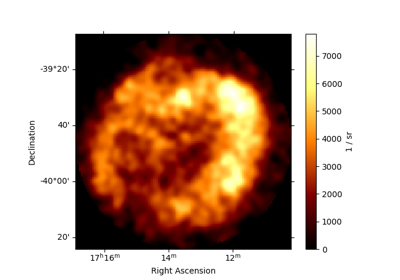Template spatial model

Template spatial model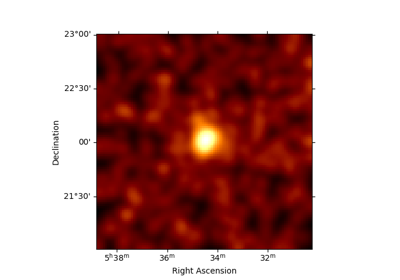High level interface

High level interface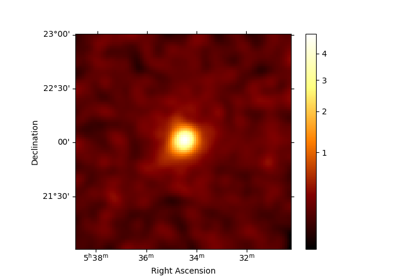Low level API

Low level API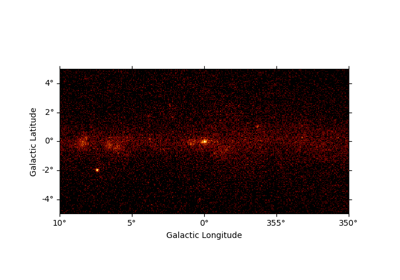Data structures

Data structures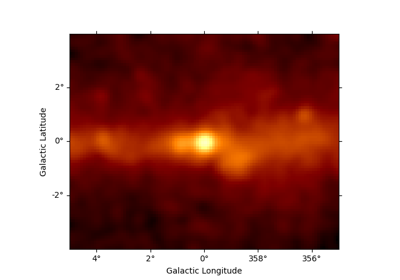Fermi-LAT with Gammapy

Fermi-LAT with Gammapy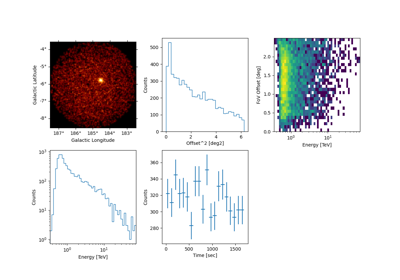H.E.S.S. with Gammapy

H.E.S.S. with Gammapy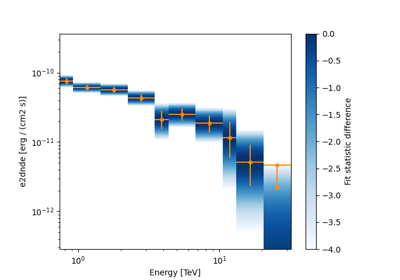Spectral analysis

Spectral analysis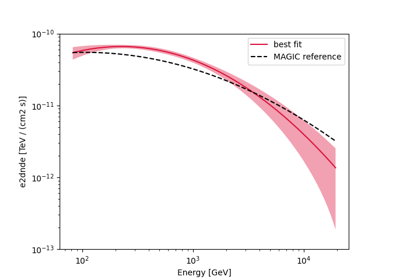Spectral analysis with energy-dependent directional cuts

Spectral analysis with energy-dependent directional cuts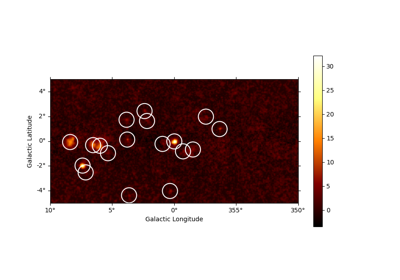Source detection and significance maps

Source detection and significance maps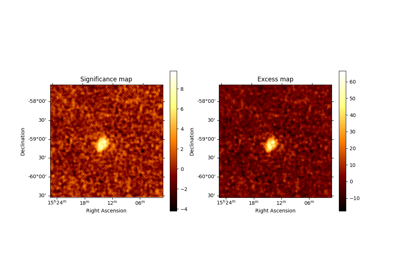Ring background map

Ring background map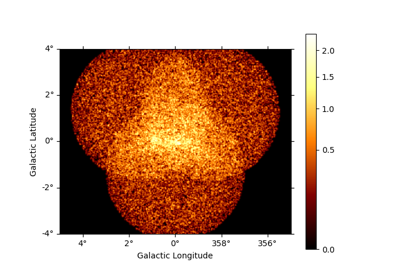3D detailed analysis

3D detailed analysis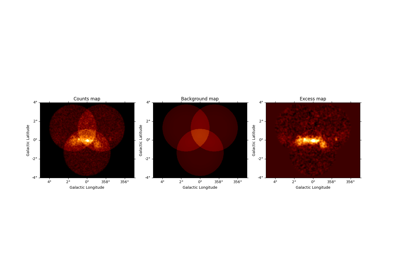Basic image exploration and fitting

Basic image exploration and fitting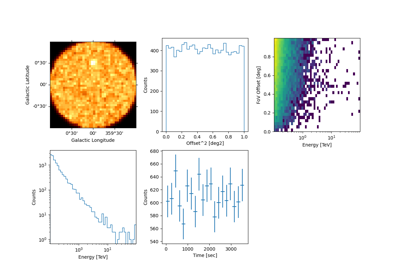Event sampling

Event sampling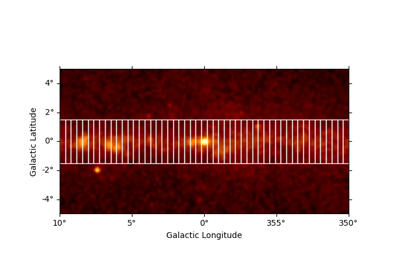Flux Profile Estimation

Flux Profile Estimation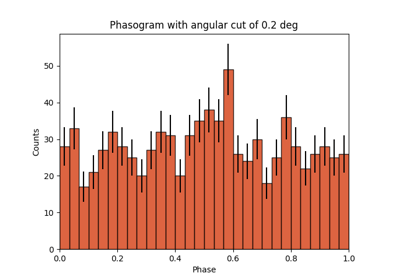Pulsar analysis

Pulsar analysis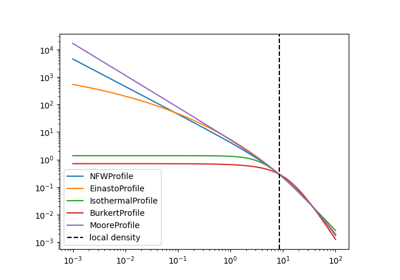Dark matter spatial and spectral models

Dark matter spatial and spectral models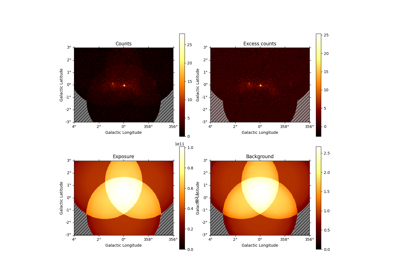Datasets - Reduced data, IRFs, models

Datasets - Reduced data, IRFs, models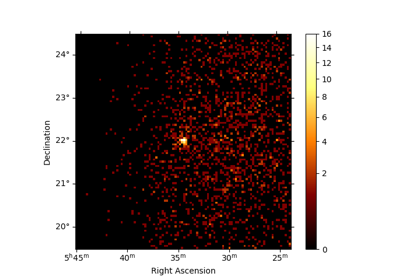Makers - Data reduction

Makers - Data reduction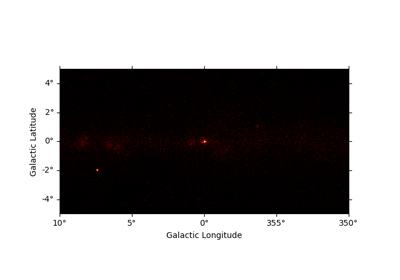Maps

Maps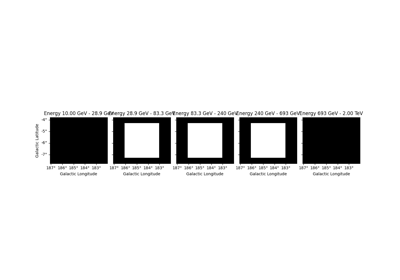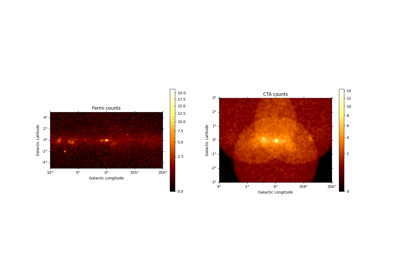Modelling

Modelling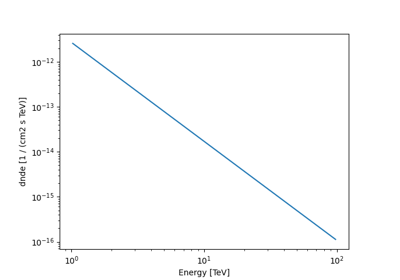Models

Models

### Examples using `gammapy.maps.RegionNDMap`#Fermi-LAT with Gammapy

Fermi-LAT with Gammapy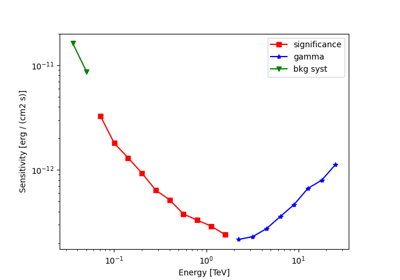Point source sensitivity

Point source sensitivitySpectral analysis with energy-dependent directional cuts

Spectral analysis with energy-dependent directional cutsPulsar analysis

Pulsar analysis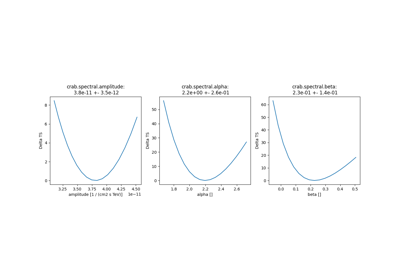Fitting

FittingMakers - Data reduction

Makers - Data reduction

### Examples using `gammapy.maps.HpxNDMap`#Fermi-LAT with Gammapy

Fermi-LAT with GammapyMaps

Maps